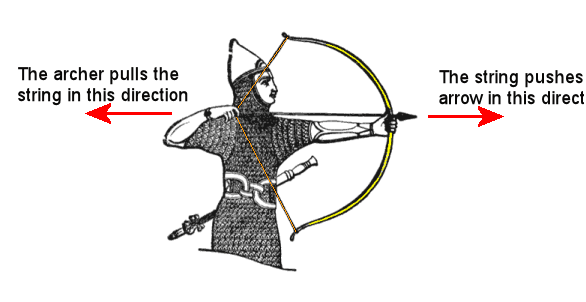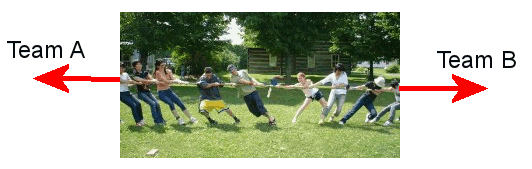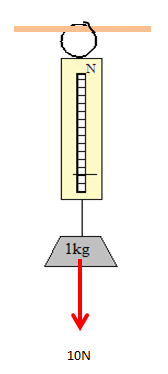[Force] [Magnets] [Friction] [Gravity and Upthrust]

A force is any kind of PUSH or PULL.

 Examples of actions that need a force: pushing a toy car to make it move squashing some modelling clay hitting a cricket ball bending a stick

Forces are needed to do the following:

• to start and stop objects moving
• to make objects change direction
• to make objects change shape

Forces cause movementForces work in pairsIf the two pulling forces are equal the teams do not move When one force is greater the teams move towards the larger force Equal forces = no change in movement Unequal forces = movement

Measuring Force

The unit of force is the newton (N).

Forces can be measured using a spring balanceUsing a spring balance to measure the pull of gravity

(A spring balance is sometimes called a force meter or Newton meter)

Types of Force

Some forces have special names:

• Magnetism: The pull or push produced by a magnet
• Gravity: The force that gives us weight. Gravity always pulls downwards
• Friction: A force that slows things down
• Upthrust: A force that makes things float

(click on each force to find out more about it)